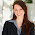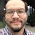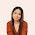## Saturday, September 8, 2012

### Time Value of Money Tips and Tricks (Real Estate)

Tips and Tricks - Time Value of Money

Here is a way to solve the following financial problems with TVM (Time Value of Money) functions:

* Finding the balance of a loan
* Finding the total amount of interest paid
* Finding the APR
* Finding the maximum loan amount based on the 28/36 ratio

Conventions and Assumptions

Variables:
N = number of months
I/YR = annual interest
i = periodical interest. This is the interest rate divided by 12. This key is present on some financial calculators instead of the I/YR key. The most famous example is the HP-12.
PV = present value.
PMT = periodic payment
FV = future value

We are assuming the following;
1. Payments are made on a monthly basis
2. The payments are due at the end of each month.
3. P/Y (payments are year) and if applicable, C/Y (compounding periods per year), are set to 12.
4. If a calculator or app is used, the normal cash flow convention applies. In our examples today, we will use the borrower's point of view. PV is positive (loan is "received"), and PMT and FV (paid out).

Let's begin!

Finding the Balance after M months.

Steps:
1. Establish the loan information. You will need, interest rate, loan amount, and payment.
2. Enter M for N and solve for FV.

Example: Find the balance on a 30-year, 5%, \$214,000 loan after 5 years (60 payments).

Step 1: Establish the loan information. At this time, payment is not known, so let's solve for it first.

N = 360
I/YR = 5
PV = 214,000
FV = 0
Solve for PMT. (-1,148.80)

Step 2: Find the balance after 60 months.

N = 60
I/YR, PV, and PMT remain unchanged.
Solve for FV. (-196,513.49)

After 60 months, the balance is \$196,513.40.

Finding the total amount of interest paid

Steps - Interest paid after the entire loan is paid:
1. Establish the loan information: term, interest rate, payment, and loan amount.
2. The interest paid is found by the formula: N × PMT + PV

A suggested keystroke sequence: RCL N × RCL PMT + RCL PV
(Remember in loans PMT is negative, PV is positive from the point of view of the borrower.)

Example: A company finances a machine costing \$5,412.50 which is financed for 3 years at 6%. The loan is carried full term. What is the total interest paid?

Step 1: Establish loan information.
N = 36
I/YR = 6
PV = 5412.50
FV = 0
Solve for PMT (-164.66)

Step 2: Find the total interest paid over the life of the loan.
PMT × N + PV = -164.66 × 36 + 5412.50 = -515.21

The company will pay a total of \$515.21 over the life of the loan.

Finding the APR

The APR, known as the annual percentage rate, is the true interest rate of the mortgage when fees, insurance, and closing costs are considered. The APR of the loan will be higher than the loan's stated rate. By law (U.S.), lending institutions must explicitly state the loan's APR.

Steps:
1. Figure out the total payment when factoring in the loan fees, insurance, and closing costs.

N = number of payments
I/YR = original rate
PV = loan amount plus loan fees plus insurance plus closing costs
FV = 0
Calculate PMT

2. Figure APR (interest rate, I/Y) with the loan amount only set as PV.

N = N from Step 1
PV = loan amount
FV = FV from Step 1
PMT = PMT from Step 1
Calculate I/Y

Example: A couple is purchasing a home costing \$300,000. The couple put 20% and will finance the balance. The loan is 30 years, financed at the rate of 4.25%. The loan charges 1 point (1% of the price) in fees and \$1,500 in mortgage insurance.

\$300,000 less the 20% down payment is \$240,000. This will be treated as the loan amount.

Step 1: Payment when factoring the fees
PV = (300,000 - 20%) + (300,000 × 1%) + 1,500.00
= 240,000 + 3,000 + 1,500 = 244,500
N = 360
I/Y = 4.25
FV = 0
Calculate PMT (-1,202.79)

The payment is \$1,202.79.

Step 2: Finding the APR
PV = 240,000
Calculate I/Y (4.41)

The APR is 4.41%.

Finding the maximum loan amount based on the 28/36 ratio.

This is how a way to find the qualifying amount for a potential borrower.

The 28/36 ratio refers to ability to pay monthly bills. On the front end, your monthly mortgage payments, which includes property taxes and insurance must be no greater than 28% of your monthly gross income. On the back end, total monthly debt payments, including the mortgage, child support, credit card, student loans, and automobile loans, must be no greater than 36%. If both ratios are met, your monthly payment is deemed qualified - you can afford it.

Using this information, we can find the maximum allowable loan amount.

Step 1: Take the lesser of:

X = .36 × monthly gross income - (monthly property insurance + monthly property taxes + other monthly debt payments)

or

X = .28 × monthly gross income - (monthly property insurance + monthly property taxes)

Step 2: Set up your TVM variables as such:
N = number of payments
I/YR = stated rate of the loan
FV = 0
PMT = -X (note the negative sign)
Solve for PV. This is your maximum loan amount.

Example: A couple wants to know the maximum price of a house they can afford in Math City. The following data is presented:
1. The couple's gross income each month is \$7,600.
2. The average property taxes and mortgage in Math City is approximately \$314 a month.
3. The couple is currently paying \$500 in credit card debt. In addition, they have financed one of their cars with a monthly payment of \$355 a month.
4. The Square Root Bank, the mortgage company the couple is currently working with, is offering a 30 year loan at 5.12%.

Step 1:
At the 36% limit, X = .36 × 7600 - (314 + 500 + 355) = 1,567
At the 28% limit, X = .28 × 7600 - 314 = 1,814
Let X = 1,567.

Step 2:
N = 360
I/YR = 5.12
FV = 0
PMT = -1567
Solve for PV (287,956.53)

The maximum loan the couple can afford is \$287,956.53.

I hope you find this helpful. Until next time,

Eddie

This blog is property of Edward Shore. © 2012

1.This comment has been removed by the author.

2.Charles,

Thanks for the compliment, and I agree that time value of money is fundamental.

Eddie

3.After reading your post, I got great learning about The Time Value of Money. That's really great information which you have shared in your post. It is very useful for those who are dealing with finance.
_______________________________________

sell a structured settlement

4.I have been searching for this information since a long time and here I finally find it. APR calculations are a tough nut to crack.

1.This a great link Wolston. Thanks for sharing!

Eddie

5.Omg! Thank you for these tips and tricks, It's really helpful and relevant. I will apply these on my daily money decisions. Can you make an article about Multiple Streams of Passive Income ? I needed your opinion about that topic. Thanks so much

6.Thanks for your post. I’ve been thinking about writing a very comparable post over the last couple of weeks, I’ll probably keep it short and sweet and link to this instead if thats cool. Thanks. convert money

### Retro Review: Radio Shack EC-2024

Retro Review:  Radio Shack EC-2024 Quick Facts Company:  Radio Shack Years in Production: Around 1994 Original Price: \$24.99 Bat...